# Gantt Charts

(diff) ← Older revision | Latest revision (diff) | Newer revision → (diff)

This mini-tutorial illustrates the use of the Bar Origin graphing role in a creative fashion to create a rudimentary Gantt chart. A Gantt chart displays a time line showing when various tasks occur in a product schedule. The use of graphing roles, and assigning graphing dimensions to graphing roles, presented by Analyica 4.0 provides a very flexible graphing framework, mastery of which allows you to present an extremely wide variety of data presentations. This tutorial provides one example that may help you develop a further mastery of graphing roles.

In the role-based graphing paradigm found in Analytica, a bar in a bar chart is a depiction of a data point. Each bar has potentially three component dimensions to it, determining where the bar is located along the horizontal axis (or vertical axis if Swap XY is on), where the bar starts (its origin), and where the bar ends (its primary value). Using graphing roles to control each of these elements, it is possible to position bars in creative ways, resulting in a wide variety of possible data presentations. The rudimentary Gantt chart is such an example, and illustrative of how these roles are controlled, as well as how multiple data to graph is assembled into a single graph.

## Components of a Gantt Chart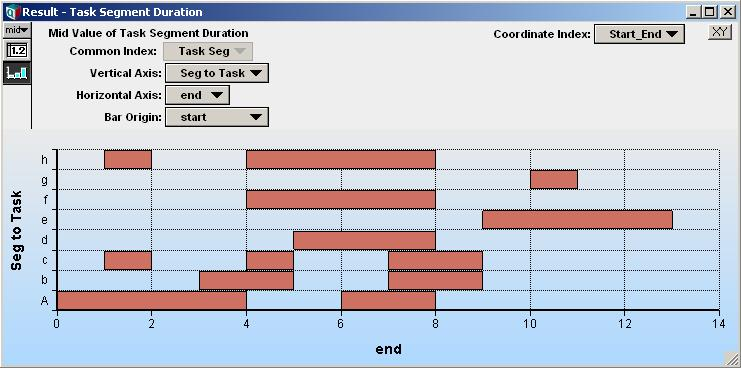A Gantt chart is composed of task segments, each segment composed of three pieces of information:

• The task that the segment belongs to.
• The start time for the task segment
• The end time for the task segment.

In the graph above, The task segment at the top left is part of task h, starts at 1 and ends at 2.

There are several ways that this data can be organized in your model. For example:

• You could have three separate variables, each indexed by Task_Seg
• You could have one two-dimensional table containing the start and end times of each task-segment, and a separate variable mapping task segment to task.
• You could have a single two-dimensional table containing three columns.

In this tutorial, we use the second arrangement, and leave the other two cases as exercises.

## Arrange the Data

To save time, I've gathered and arranged the data for you already to provide a starting point.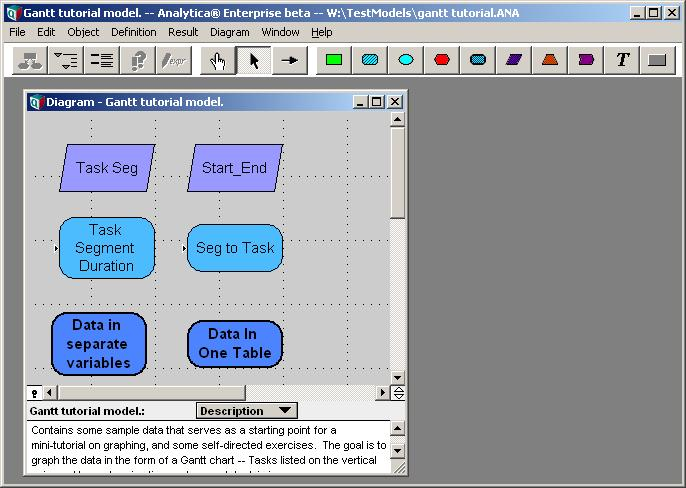This model contains the data that we will use for graphing. If this were a real modeling application, the data here would probably be computed based on various modeling assumptions and projections. Since this mini-tutorial focuses on graphing concepts, I've provided pre-canned data to work with.

3. Show the result table for Task_Segment_Duration.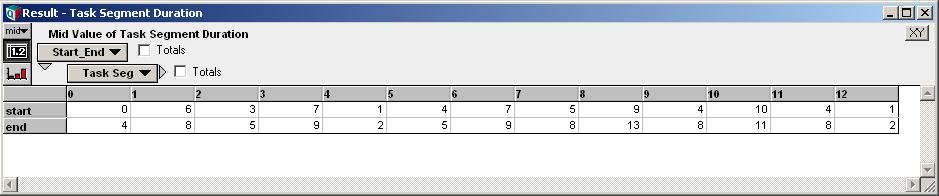Here we have the start and end times for each task segment. For example task segment 6 starts on day 7 and ends on day 9.

From the graphing perspective, here is the important thing to observe about this data. We want each of the rows shown in this table to serve a separate role in the graph we are about to create. The start should determine the left-edge of the bar (the bar-origin role), and the end should determine the right-edge of the bar when each bar is plotted. We can view each column of this table as providing a graphing coordinate -- two numbers specifying (or partially specifying) how a data point (in this case a bar segment is the data point) is to be displayed on the graph. We will call the index, Start_End, a coordinate index, since it indexes each graphing coordinate vector.

4. Close that window and show the result table for Seg_to_task.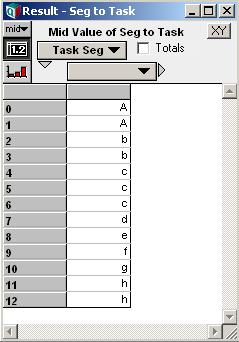Here you can see how each task segment is mapped to its task. We've used the letters 'A' through 'H' as task names.

The task here ('A' through 'H') will serve as one additional component of the graphing coordinate, in this case determining where along the Y-axis the bar should be displayed.

5. Close this result window.

## Selecting Graphing Dimension Data

To create the Gantt chart, we are going to use the data contained in both these variables in a single graph. We'll use the columns of Task_Segment_Duration, and bring Seg_to_task in as an external graphing dimension.

6. Show the result for Task_Segment_Duration (again), and switch to graph mode. Initially the graph displays as: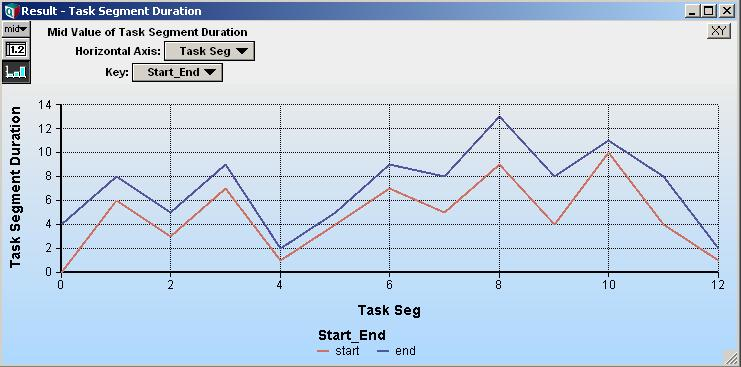7. Next, bring in the Task_to_seg result as an external variable. To do this, click on the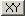at the top right of the result window. If you don't see this button, you are in browse mode and must first click the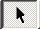toolbar button to enter edit mode.

8. In the XY coordinate sources dialog, check both checks, Use coordinate index and Use another variable or expression, then press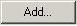and select Seg_to_task.We are using a coordinate index here because we want to treat the two rows of Task_segment_duration as separate graphing dimensions. One of these rows will be used for the left edge of the bar (the bar origin), and the other row will be used for the right edge of the bar (the y-axis role). Hence, we have checked Use coordinate index.

We have also brought in one external graphing dimension, Seg_to_task. This contains the task for each segment. The task for each segment will determine which row along the vertical axis the corresponding bar is plotted in.

9. Press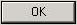twice to close the dialogs.

Notice that a Coordinate Index pulldown has appeared above the graph. Since we want the Start_End index to be our coordinate index (see comments at Step 3), select Start_End in that pulldown.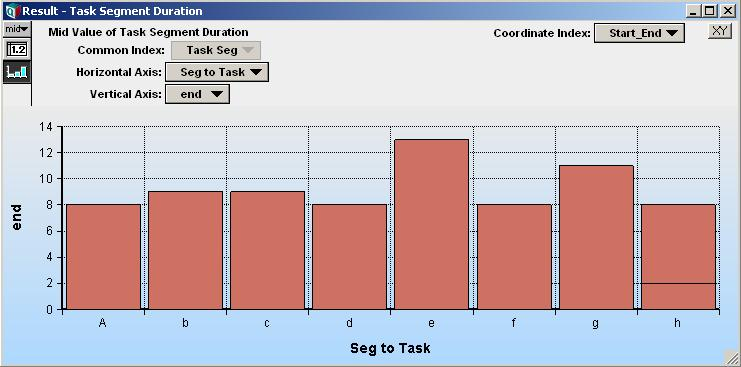10. Pressto view the result table.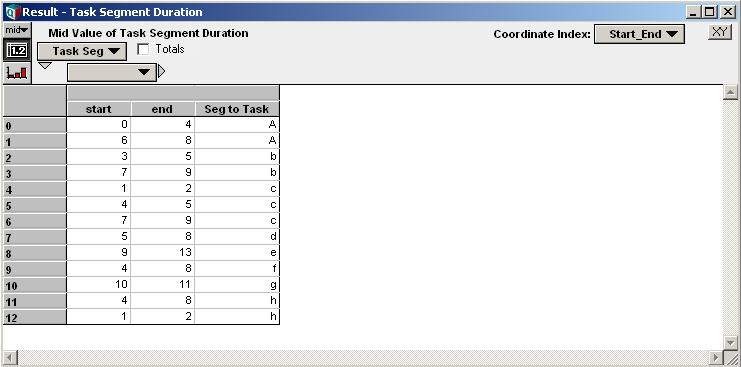The table shows that the data has been gathered and arranged into twelve data points, each point being a vector of three elements. The second row of the column header displays the names of these three elements.

11. Pressto return to the result graph.

## Using the Bar Origin role

Normally, bars in a bar chart all start at the origin and extend to the primary value. In this example, we want the beginning of each bar to reflect the task start value. To enable selection of a non-standard bar-origin, you must indicate so in the graph setup dialog.

12. Double click on the graph to bring up the Graph Setup dialog. The Bar chart line style should already selected (if not, select it), then click the checkbox for "Variable origin (e.g., tornado plot)":(Note: The text for this option may change. If you do not see this exact text, you should be able to quickly identify the renamed option).

Checking this option also selected the Swap horizontal and vertical checkbox, which we want as well. Swapping axes displays horizontal, rather than vertical, bars.

13. Press Apply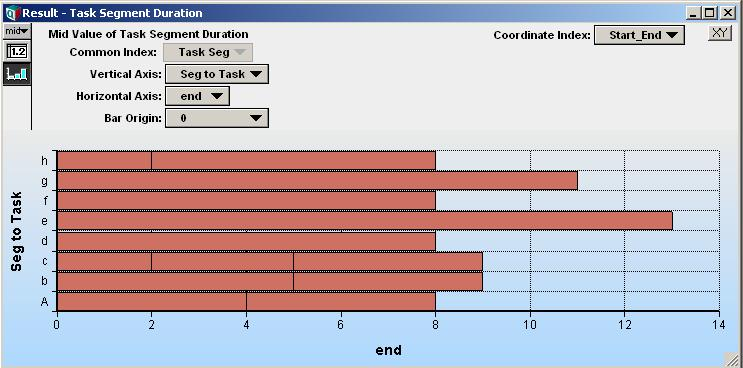14. Now use the Bar Origin pulldown above the graph to select start for the bar origin. The pulldowns should be as shown in the following screenshot (if not, change them to be so):This provides the basic Gantt chart.

Notice that the Vertical Axis role is set to Seg to Task. This is controlling the placement of each bar, so that each bar is drawn in the column corresponding to its task.

## Further Exercises

### Exercise 1

In the module, Data in separate variables, the three components of a Gantt chart are organized in three separate variables. In the result graph for Seg_end, produce the equivalent Gantt chart.

In the module, Data in One Table, all three components of the Gantt chart are organized in a single table with 3 columns. Configure the graph for Add_data to display the Gantt chart.